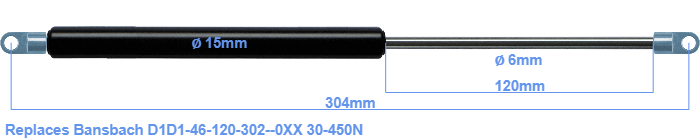# Replacement for Bansbach D1D1-46-120-302–0XX 30-450N

£27.15 (excl. VAT)

Replacement gas spring for the Bansbach D1D1-46-120-302--0XX 30-450 Newton. The eye has a thickness of 3mm and a hole diameter of 6.1mm. Brand: Hahn.
Force Choose an option30 Newton40 Newton50 Newton60 Newton80 Newton100 Newton120 Newton140 Newton150 Newton160 Newton180 Newton200 Newton220 Newton240 Newton250 Newton260 Newton280 Newton300 Newton320 Newton340 Newton350 Newton360 Newton380 Newton400 Newton420 Newton440 Newton450 NewtonClear